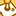# New to Qlik Sense

If you’re new to Qlik Sense, start with this Discussion Board and get up-to-speed quickly.

Announcements
cancel
Showing results for
Did you mean:Contributor III

## Variable calculating over whole dataset, not by row

Hello,

I have a variable with the following expression:

This formula works fine when I add this to a measure but if I add this to a variable and use the variable as the measure, the sum is adding the entire dataset, not the row only.

How do I correct this?

Thanks

1 Solution

Accepted SolutionsMVP

Hi Ken,

The reason is the equal sign "=" in the beginning of the formula. Variables that contain formulas, beginning with an equal sign, are calculated after every change in the data set (selections, changes in other variables, etc...)

The same formula stored in a variable without the beginning equal sign, will produce accurate results when used in a Measure, being enclosed in a \$-sign expansion, like this:

\$(myFormula)

Best,

3 RepliesMVP

Hi Ken,

The reason is the equal sign "=" in the beginning of the formula. Variables that contain formulas, beginning with an equal sign, are calculated after every change in the data set (selections, changes in other variables, etc...)

The same formula stored in a variable without the beginning equal sign, will produce accurate results when used in a Measure, being enclosed in a \$-sign expansion, like this:

\$(myFormula)

Best,Contributor III
Author

Hi Oleg,

I have now run into a new problem as part of this same sheet. I have a variable vQty_0% with the following expression:

if(vUsage_1 < [Qty On Hand], vUsage_1, [Qty On Hand])

This formula is giving me the else outcome even through the true condition is being met. vUsage_1 = 51 & [Qty on hand] = 2171. I have checked the format of Qty on hand and this is a numerical field so should be able to compare against each other.

Any ideas on how to resolve?

Thanks

KenContributor III
Author

I figured this one out. I needed to add the \$ sign and brackets around the variable.

if(\$(vUsage_1) < [Qty On Hand], \$(vUsage_1), [Qty On Hand])